## 6.2.1 Representation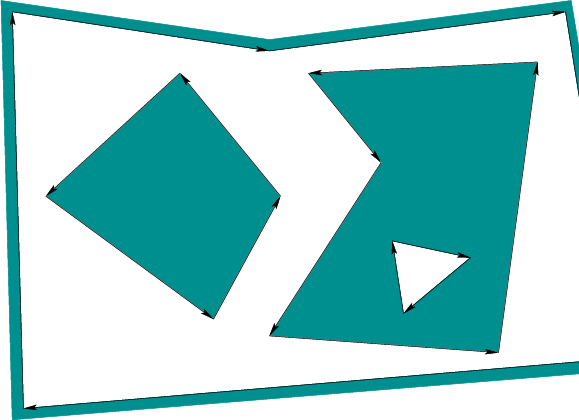Assume that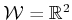; the obstacles,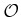, are polygonal; and the robot,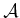, is a polygonal body that is only capable of translation. Under these assumptions,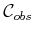will be polygonal. For the special case in whichis a point in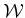,maps directly towithout any distortion. Thus, the problems considered in this section may also be considered as planning for a point robot. Ifis not a point robot, then the Minkowski difference, (4.37), ofandmust be computed. For the case in which bothand each component ofare convex, the algorithm in Section 4.3.2 can be applied to compute each component of. In general, bothandmay be nonconvex. They may even contain holes, which results in amodel such as that shown in Figure 6.1. In this case,andmay be decomposed into convex components, and the Minkowski difference can be computed for each pair of components. The decompositions into convex components can actually be performed by adapting the cell decomposition algorithm that will be presented in Section 6.2.2. Once the Minkowski differences have been computed, they need to be merged to obtain a representation that can be specified in terms of simple polygons, such as those in Figure 6.1. An efficient algorithm to perform this merging is given in Section 2.4 of . It can also be based on many of the same principles as the planning algorithm in Section 6.2.2.

To implement the algorithms described in this section, it will be helpful to have a data structure that allows convenient access to the information contained in a model such as Figure 6.1. How is the outer boundary represented? How are holes inside of obstacles represented? How do we know which holes are inside of which obstacles? These questions can be efficiently answered by using the doubly connected edge list data structure, which was described in Section 3.1.3 for consistent labeling of polyhedral faces. We will need to represent models, such as the one in Figure 6.1, and any other information that planning algorithms need to maintain during execution. There are three different records:

1. [] Vertices: Every vertex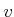contains a pointer to a point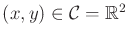and a pointer to some half-edge that hasas its origin.
2. [] Faces: Every face has one pointer to a half-edge on the boundary that surrounds the face; the pointer value is NIL if the face is the outermost boundary. The face also contains a list of pointers for each connected component (i.e., hole) that is contained inside of that face. Each pointer in the list points to a half-edge of the component's boundary.
3. [] Half-edges: Each half-edge is directed so that the obstacle portion is always to its left. It contains five different pointers. There is a pointer to its origin vertex. There is a twin half-edge pointer, which may point to a half-edge that runs in the opposite direction (see Section 3.1.3). If the half-edge borders an obstacle, then this pointer is NIL. Half-edges are always arranged in circular chains to form the boundary of a face. Such chains are oriented so that the obstacle portion (or a twin half-edge) is always to its left. Each half-edge stores a pointer to its internal face. It also contains pointers to the next and previous half-edges in the circular chain of half-edges.
For the example in Figure 6.1, there are four circular chains of half-edges that each bound a different face. The face record of the small triangular hole points to the obstacle face that contains the hole. Each obstacle contains a pointer to the face represented by the outermost boundary. By consistently assigning orientations to the half-edges, circular chains that bound an obstacle always run counterclockwise, and chains that bound holes run clockwise. There are no twin half-edges because all half-edges bound part of. The doubly connected edge list data structure is general enough to allow extra edges to be inserted that slice through. These edges will not be on the border of, but they can be managed using twin half-edge pointers. This will be useful for the algorithm in Section 6.2.2.

Steven M LaValle 2020-08-14